Search
 Return to the Lessons Index  | Do the Lessons in Order  |  Print-friendly page

Complex Fractions: Technicalities

I started with:

• Simplify the following expression:
•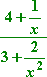...and ended up with: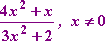To get this "x not equal to zero" restriction, I had to consider all of the denominators, both of the entire fraction and of the sub-fractions. The two sub-fractions were the 1/x and the 2/x2 in the numerator and denominator:   Copyright © Elizabeth Stapel 2003-2011 All Rights Reserved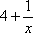and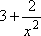Each of these expressions is undefined if x = 0.

Then I also have to consider the denominator of the whole complex fraction. Recall that this was rearranged to be: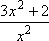A fraction is zero when its numerator is zero, so the above fraction would make the complex fraction undefined when 3x2 + 2 = 0. However, this is never equal to zero, so I got no further restrictions on my answer.

Note: If you're going to have to find these restrictions on your answers, you may want to stick with the "flip-n-multiply" method of simplification, instead of the "multiply through by the common denominator" method, since you'll need to convert the complex fraction's denominator into a fraction anyway. If you don't have to find these restrictions, be grateful, and don't worry about these notes on the rest of the examples in this lesson.

Return to the lesson

Top  |  Return to Index

Study Skills Survey

Tutoring from Purplemath
Find a local math tutor

 Copyright © 2021  Elizabeth Stapel   |   About   |   Terms of Use   |   Linking   |   Site Licensing Contact Us